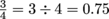Study Guide

# Ratios & Percentages - Comparing Percents, Fractions, & Decimals

## Comparing Percents, Fractions, & Decimals

What should we do if we find two or more of these forms mixed together in a sentence or a math problem? That's like comparing apples and oranges and dragonfruits, isn't it?

Don't sweat it. Just use deodorant and convert! We can use whatever form is easiest at the moment—percents, fractions, or decimals.

Say we're asked to write these values in order from least to greatest:, 0.72, and 43%. To do that, we first need to write them all in the same format.

It's as hard to compare fractions to decimals as it is to compare apples to grapefruit. So let's convert each number to a decimal and then compare them.0.72 = 0.72
43% = 0.43 (after moving the decimal left by two places)

Now we can see that the smallest value is 0.43 or 43%. The next is 0.72, and the largest is 0.75 or.

We can technically convert all three numbers into whatever form we want, but it's usually easiest to turn them all into decimals. Just FYI.